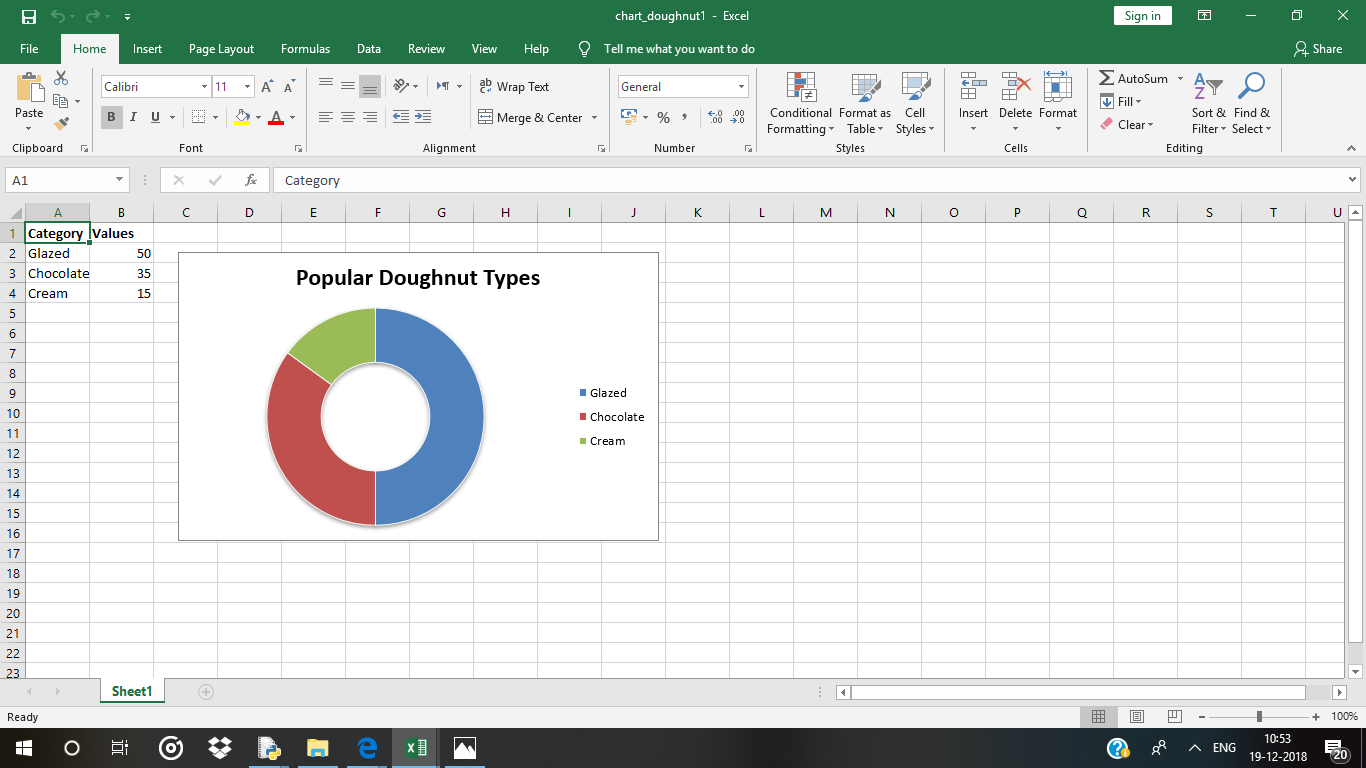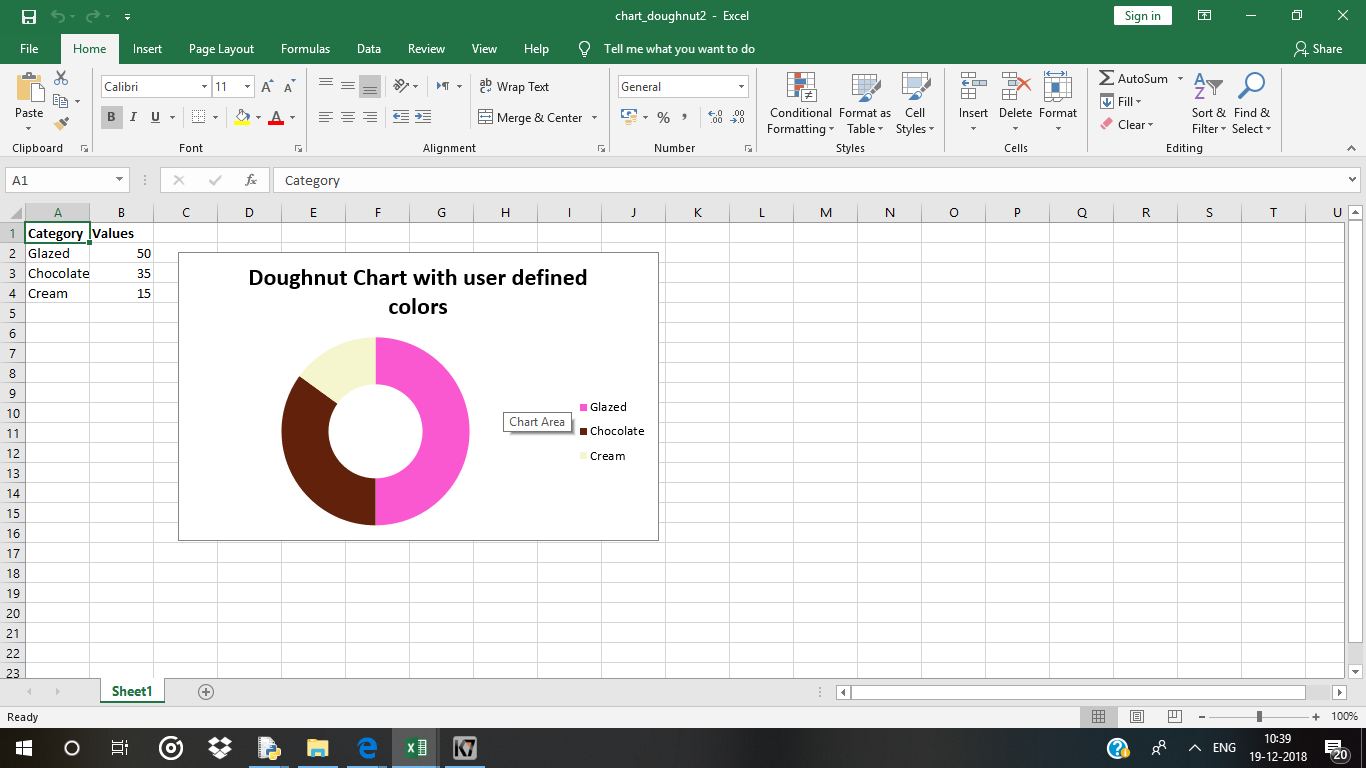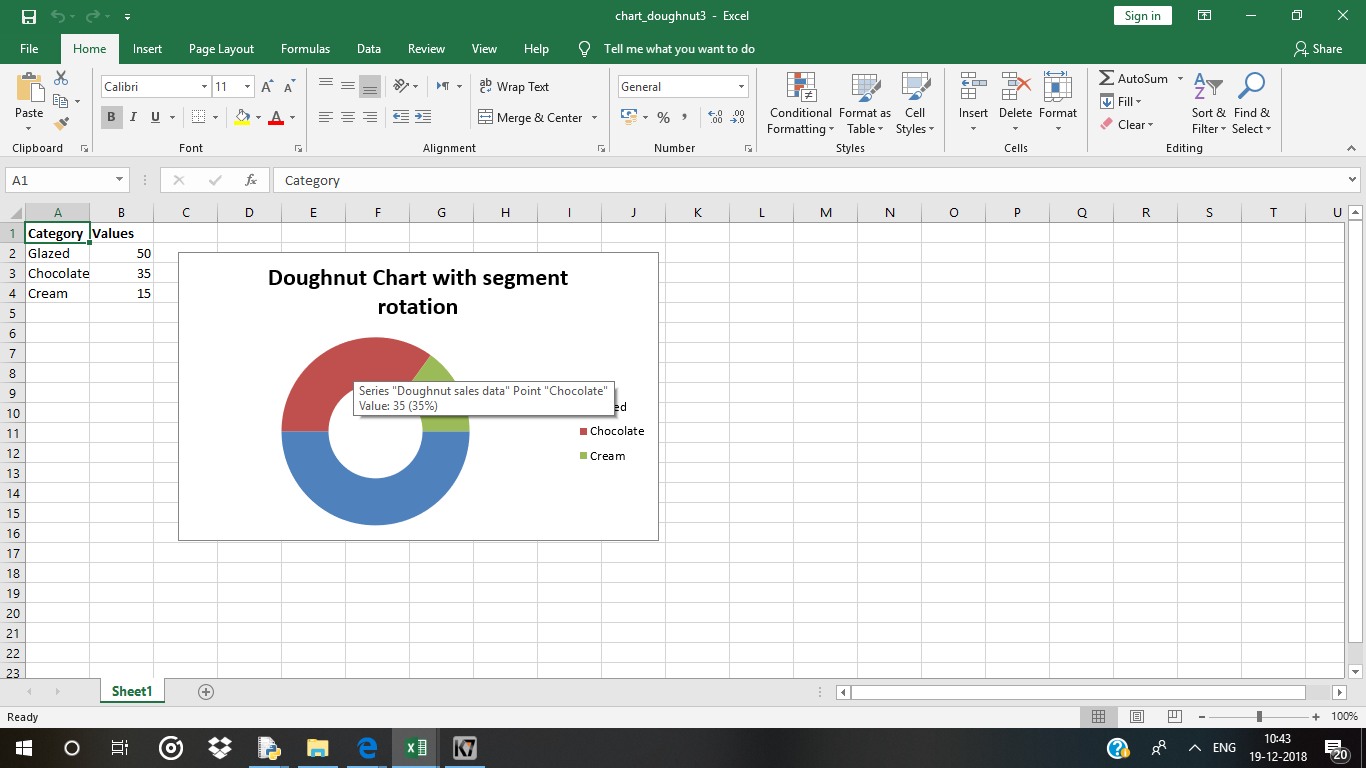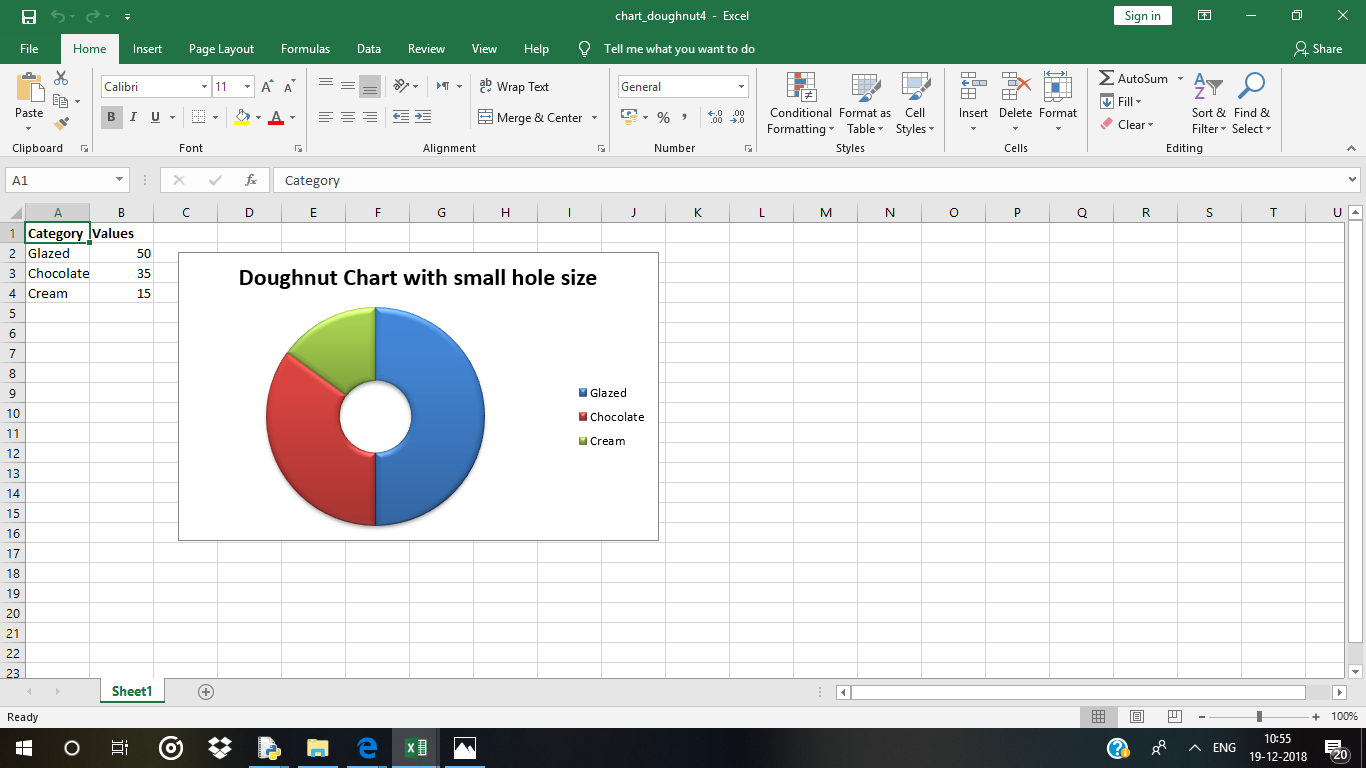# Python | Plotting Doughnut charts in excel sheet using XlsxWriter module

Prerequisite: Create and Write on an excel sheet

`XlsxWriter` is a Python library using which one can perform multiple operations on excel files like creating, writing, arithmetic operations and plotting graphs. Let’s see how to plot different variety of doughnut charts using realtime data.

Charts are composed of at least one series of one or more data points. Series themselves are comprised of references to cell ranges. For plotting the charts on an excel sheet, firstly, create chart object of specific chart type( i.e Doughnut chart etc.). After creating chart objects, insert data in it and lastly, add that chart object in the sheet object.

Code #1 : Plot the simple Doughnut Chart.

For plotting the simple Pie chart on an excel sheet, use `add_chart() `method with type ‘doughnut’ keyword argument of a workbook object.

 `# import xlsxwriter module  ` `import` `xlsxwriter ` ` `  `# Workbook() takes one, non-optional, argument    ` `# which is the filename that we want to create.  ` `workbook ``=` `xlsxwriter.Workbook(``'chart_doughnut1.xlsx'``) ` ` `  `# The workbook object is then used to add new    ` `# worksheet via the add_worksheet() method.   ` `worksheet ``=` `workbook.add_worksheet() ` ` `  `  `  `# Create a new Format object to formats cells  ` `# in worksheets using add_format() method .  ` `   `  `# here we create bold format object .  ` `bold ``=` `workbook.add_format({``'bold'``: ``1``}) ` ` `  `# Add the worksheet data that the charts will refer to. ` `headings ``=` `[``'Category'``, ``'Values'``] ` `data ``=` `[ ` `    ``[``'Glazed'``, ``'Chocolate'``, ``'Cream'``], ` `    ``[``50``, ``35``, ``15``], ` `] ` ` `  ` `  `# Write a row of data starting from 'A1'  ` `# with bold format . ` `worksheet.write_row(``'A1'``, headings, bold) ` ` `  `# Write a column of data starting from   ` `# 'A2', 'B2' respectively .  ` `worksheet.write_column(``'A2'``, data[``0``]) ` `worksheet.write_column(``'B2'``, data[``1``]) ` ` `  `# Create a chart object that can be added  ` `# to a worksheet using add_chart() method.  ` ` `  `# here we create a doughnut chart object .  ` `chart1 ``=` `workbook.add_chart({``'type'``: ``'doughnut'``}) ` ` `  `# Add a data series to a chart  ` `# using add_series method. ` ` `  `# Configure the first series. ` `# syntax to define ranges  ` `# [sheetname, first_row, first_col, last_row, last_col]. ` `chart1.add_series({ ` `    ``'name'``:       ``'Doughnut sales data'``, ` `    ``'categories'``: [``'Sheet1'``, ``1``, ``0``, ``3``, ``0``], ` `    ``'values'``:     [``'Sheet1'``, ``1``, ``1``, ``3``, ``1``], ` `}) ` ` `  `# Add a chart title  ` `chart1.set_title({``'name'``: ``'Popular Doughnut Types'``}) ` ` `  `# Set an Excel chart style. Colors ` `# with white outline and shadow. ` `chart1.set_style(``10``) ` ` `  `# add chart to the worksheet with an offset,  ` `# at the top-left corner of a chart   ` `# is anchored to cell C2 . ` `worksheet.insert_chart(``'C2'``, chart1, {``'x_offset'``: ``25``, ``'y_offset'``: ``10``}) ` ` `  `# Finally, close the Excel file   ` `# via the close() method.   ` `workbook.close() `

Output :Code #2 : Plot a Doughnut chart with user defined segment colors.

For plotting the pie chart with user defined segment colours on an excel sheet, use `add_series()` method with points keyword argument of a chart object.

 `# import xlsxwriter module  ` `import` `xlsxwriter ` ` `  `# Workbook() takes one, non-optional, argument    ` `# which is the filename that we want to create.  ` `workbook ``=` `xlsxwriter.Workbook(``'chart_doughnut2.xlsx'``) ` ` `  `# The workbook object is then used to add new    ` `# worksheet via the add_worksheet() method.   ` `worksheet ``=` `workbook.add_worksheet() ` ` `  `  `  `# Create a new Format object to formats cells  ` `# in worksheets using add_format() method .  ` `   `  `# here we create bold format object .  ` `bold ``=` `workbook.add_format({``'bold'``: ``1``}) ` ` `  `# Add the worksheet data that the charts will refer to. ` `headings ``=` `[``'Category'``, ``'Values'``] ` `data ``=` `[ ` `    ``[``'Glazed'``, ``'Chocolate'``, ``'Cream'``], ` `    ``[``50``, ``35``, ``15``], ` `] ` ` `  ` `  `# Write a row of data starting from 'A1'  ` `# with bold format . ` `worksheet.write_row(``'A1'``, headings, bold) ` ` `  `# Write a column of data starting from   ` `# 'A2', 'B2' respectively .  ` `worksheet.write_column(``'A2'``, data[``0``]) ` `worksheet.write_column(``'B2'``, data[``1``]) ` ` `  `# Create a chart object that can be added  ` `# to a worksheet using add_chart() method.  ` ` `  `# here we create a doughnut chart object . ` `chart2 ``=` `workbook.add_chart({``'type'``: ``'doughnut'``}) ` ` `  `# Note use of alternative syntax to define ranges. ` `# = Sheet1 !\$A\$1 is equivalent to ['Sheet1', 0, 0].  ` `# Configure the series and add user defined segment colors. ` `chart2.add_series({ ` `    ``'name'``: ``'Doughnut sales data'``, ` `    ``'categories'``: ``'= Sheet1 !\$A\$2:\$A\$4'``, ` `    ``'values'``:     ``'= Sheet1 !\$B\$2:\$B\$4'``, ` `    ``'points'``: [ ` `        ``{``'fill'``: {``'color'``: ``'# FA58D0'``}}, ` `        ``{``'fill'``: {``'color'``: ``'# 61210B'``}}, ` `        ``{``'fill'``: {``'color'``: ``'# F5F6CE'``}}, ` `    ``], ` `}) ` ` `  `# Add a chart title ` `chart2.set_title({``'name'``: ``'Doughnut Chart with user defined colors'``}) ` ` `  `# add chart to the worksheet with an offset,  ` `# at the top-left corner of a chart   ` `# is anchored to cell C2 . ` `worksheet.insert_chart(``'C2'``, chart2, {``'x_offset'``: ``25``, ``'y_offset'``: ``10``}) ` ` `  `# Finally, close the Excel file   ` `# via the close() method.   ` `workbook.close() `

Output :Code #3 : Plot a Doughnut chart with rotation of the segments.

For plotting a pie chart with rotation of the segments on an excel sheet, use `set_rotation()` method with definite angle argument of the chart object.

 `# import xlsxwriter module  ` `import` `xlsxwriter ` ` `  `# Workbook() takes one, non-optional, argument    ` `# which is the filename that we want to create.  ` `workbook ``=` `xlsxwriter.Workbook(``'chart_doughnut3.xlsx'``) ` ` `  `# The workbook object is then used to add new    ` `# worksheet via the add_worksheet() method.   ` `worksheet ``=` `workbook.add_worksheet() ` ` `  `# Create a new Format object to formats cells  ` `# in worksheets using add_format() method .  ` `   `  `# here we create bold format object .  ` `bold ``=` `workbook.add_format({``'bold'``: ``1``}) ` ` `  `# Add the worksheet data that the charts will refer to. ` `headings ``=` `[``'Category'``, ``'Values'``] ` `data ``=` `[ ` `    ``[``'Glazed'``, ``'Chocolate'``, ``'Cream'``], ` `    ``[``50``, ``35``, ``15``], ` `] ` ` `  `# Write a row of data starting from 'A1'  ` `# with bold format . ` `worksheet.write_row(``'A1'``, headings, bold) ` ` `  `# Write a column of data starting from   ` `# 'A2', 'B2' respectively .  ` `worksheet.write_column(``'A2'``, data[``0``]) ` `worksheet.write_column(``'B2'``, data[``1``]) ` ` `  `# Create a chart object that can be added  ` `# to a worksheet using add_chart() method.  ` ` `  `# here we create a doughnut chart object . ` `chart3 ``=` `workbook.add_chart({``'type'``: ``'doughnut'``}) ` ` `  `# Configure the series. ` `chart3.add_series({ ` `    ``'name'``: ``'Doughnut sales data'``, ` `    ``'categories'``: ``'= Sheet1 !\$A\$2:\$A\$4'``, ` `    ``'values'``:     ``'= Sheet1 !\$B\$2:\$B\$4'``, ` `}) ` ` `  `# Add a chart title. ` `chart3.set_title({``'name'``: ``'Doughnut Chart with segment rotation'``}) ` ` `  `# Change the angle / rotation of the first segment. ` `chart3.set_rotation(``90``) ` ` `  `# add chart to the worksheet with an offset,  ` `# at the top-left corner of a chart   ` `# is anchored to cell C2 . ` `worksheet.insert_chart(``'C2'``, chart3, {``'x_offset'``: ``25``, ``'y_offset'``: ``10``}) ` ` `  `# Finally, close the Excel file   ` `# via the close() method.   ` `workbook.close() `

Output :Code #4 : Plot a Doughnut chart with user defined hole size.

For plotting a pie chart with user defined hole size on an excel sheet, use `set_hole_size()` method with definite value(radius) argument of the chart object.

 `# import xlsxwriter module  ` `import` `xlsxwriter ` ` `  `# Workbook() takes one, non-optional, argument    ` `# which is the filename that we want to create.  ` `workbook ``=` `xlsxwriter.Workbook(``'chart_doughnut4.xlsx'``) ` ` `  `# The workbook object is then used to add new    ` `# worksheet via the add_worksheet() method.   ` `worksheet ``=` `workbook.add_worksheet() ` ` `  `  `  `# Create a new Format object to formats cells  ` `# in worksheets using add_format() method .  ` `   `  `# here we create bold format object .  ` `bold ``=` `workbook.add_format({``'bold'``: ``1``}) ` ` `  `# Add the worksheet data that the charts will refer to. ` `headings ``=` `[``'Category'``, ``'Values'``] ` `data ``=` `[ ` `    ``[``'Glazed'``, ``'Chocolate'``, ``'Cream'``], ` `    ``[``50``, ``35``, ``15``], ` `] ` ` `  ` `  `# Write a row of data starting from 'A1'  ` `# with bold format . ` `worksheet.write_row(``'A1'``, headings, bold) ` ` `  `# Write a column of data starting from   ` `# 'A2', 'B2' respectively .  ` `worksheet.write_column(``'A2'``, data[``0``]) ` `worksheet.write_column(``'B2'``, data[``1``]) ` ` `  `# Create a chart object that can be added  ` `# to a worksheet using add_chart() method.  ` ` `  `# here we create a doughnut chart object . ` `chart4 ``=` `workbook.add_chart({``'type'``: ``'doughnut'``}) ` ` `  `# Configure the series. ` `chart4.add_series({ ` `    ``'name'``: ``'Doughnut sales data'``, ` `    ``'categories'``: ``'= Sheet1 !\$A\$2:\$A\$4'``, ` `    ``'values'``:     ``'= Sheet1 !\$B\$2:\$B\$4'``, ` `}) ` ` `  `# Set a 3D style. ` `chart4.set_style(``26``) ` ` `  `# Add a title. ` `chart4.set_title({``'name'``: ``'Doughnut Chart with small hole size'``}) ` ` `  `# Change the hole size. ` `chart4.set_hole_size(``33``) ` ` `  `# Insert the chart into the worksheet (with an offset). ` `worksheet.insert_chart(``'C2'``, chart4, {``'x_offset'``: ``25``, ``'y_offset'``: ``10``}) ` ` `  `# Finally, close the Excel file   ` `# via the close() method.   ` `workbook.close() `

Output :My Personal Notes arrow_drop_upCheck out this Author's contributed articles.

If you like GeeksforGeeks and would like to contribute, you can also write an article using contribute.geeksforgeeks.org or mail your article to contribute@geeksforgeeks.org. See your article appearing on the GeeksforGeeks main page and help other Geeks.

Please Improve this article if you find anything incorrect by clicking on the "Improve Article" button below.

Article Tags :

29

Please write to us at contribute@geeksforgeeks.org to report any issue with the above content.